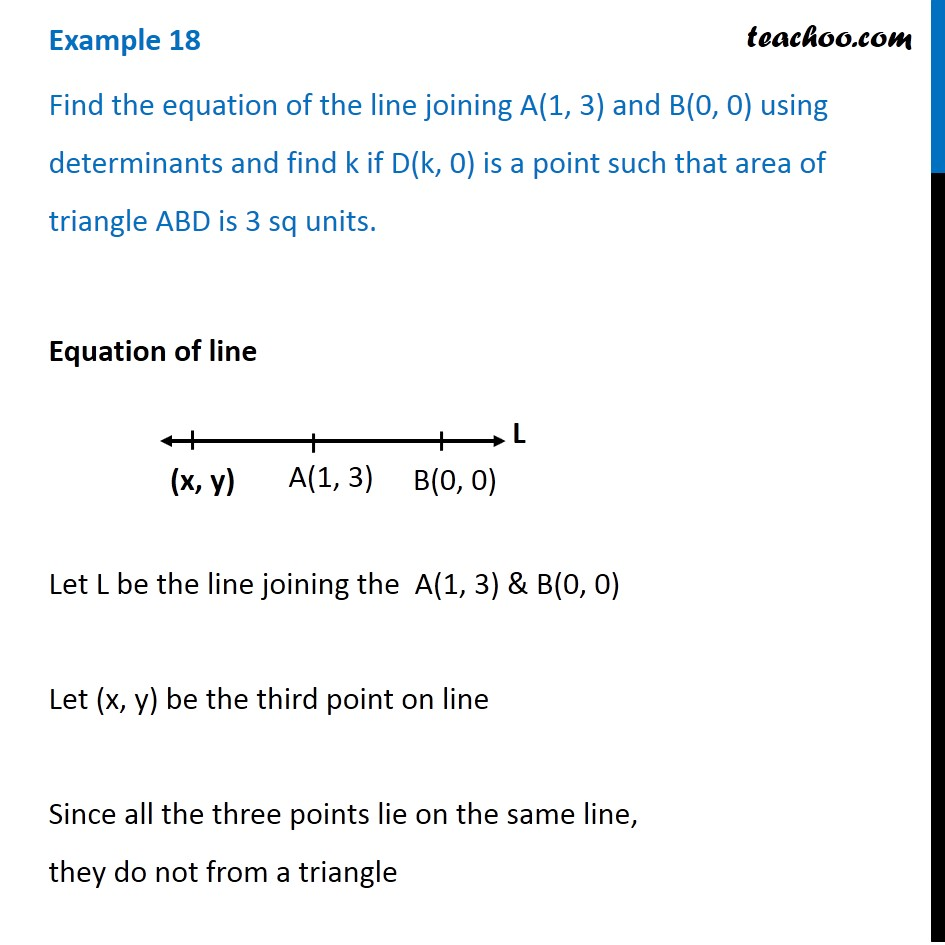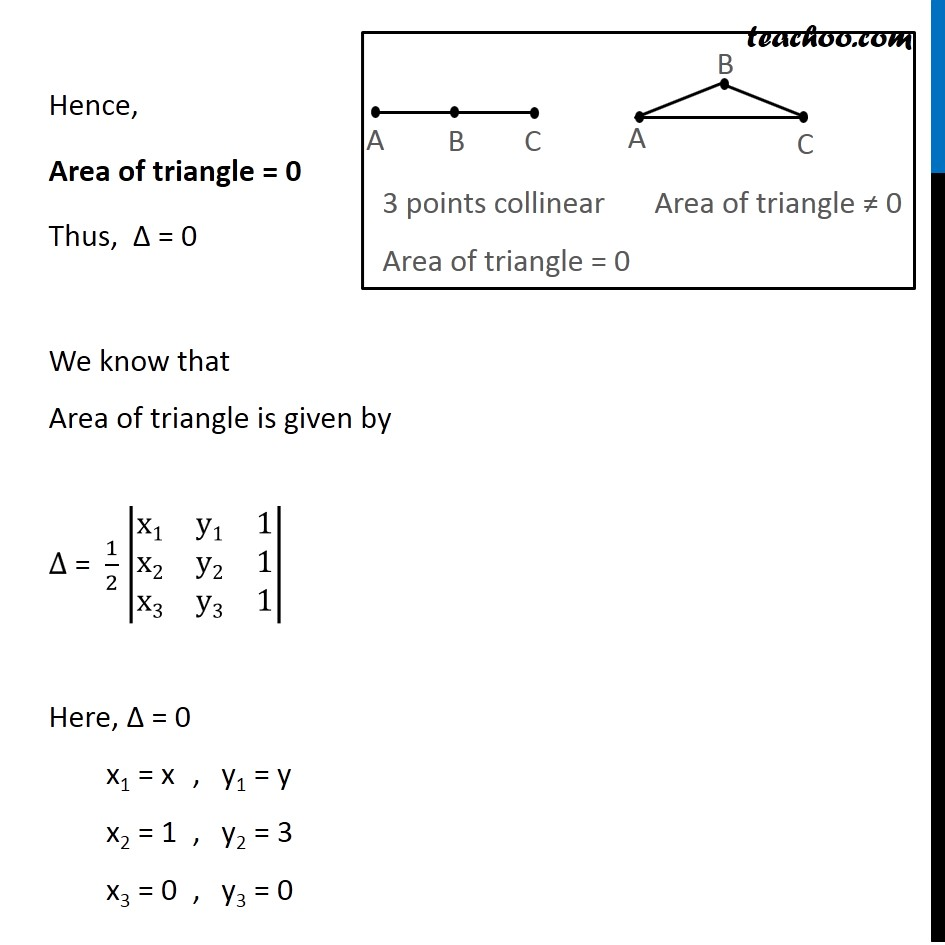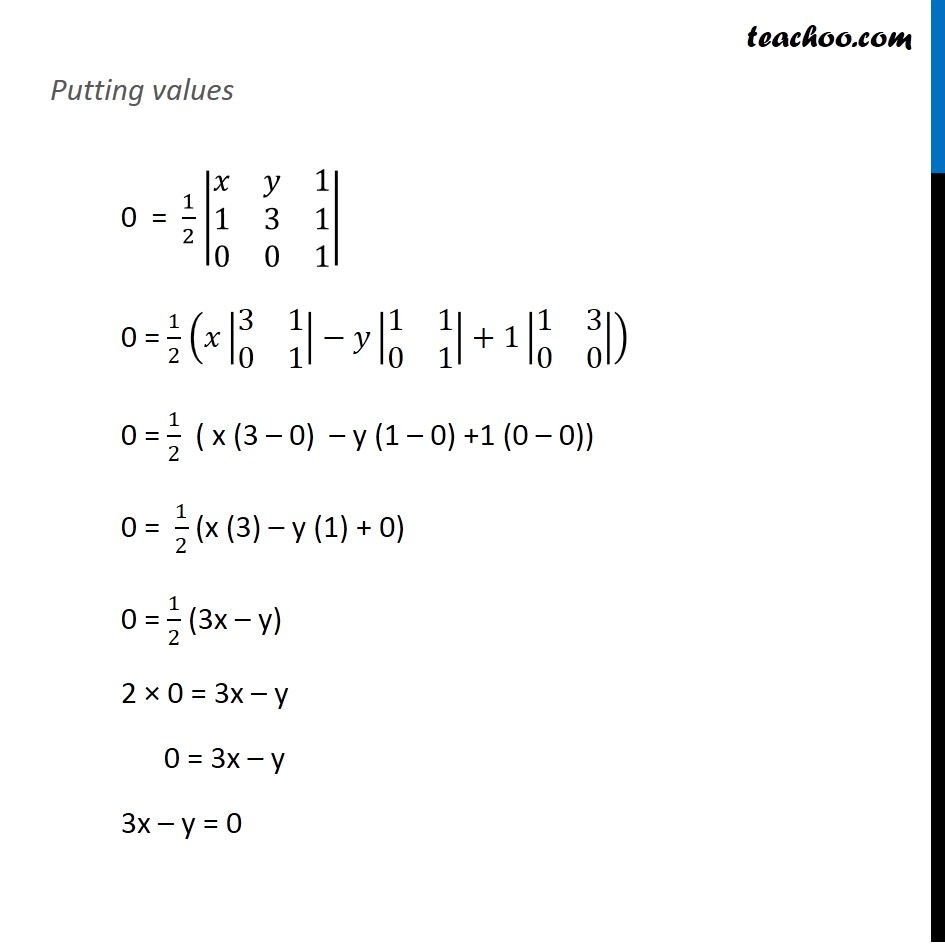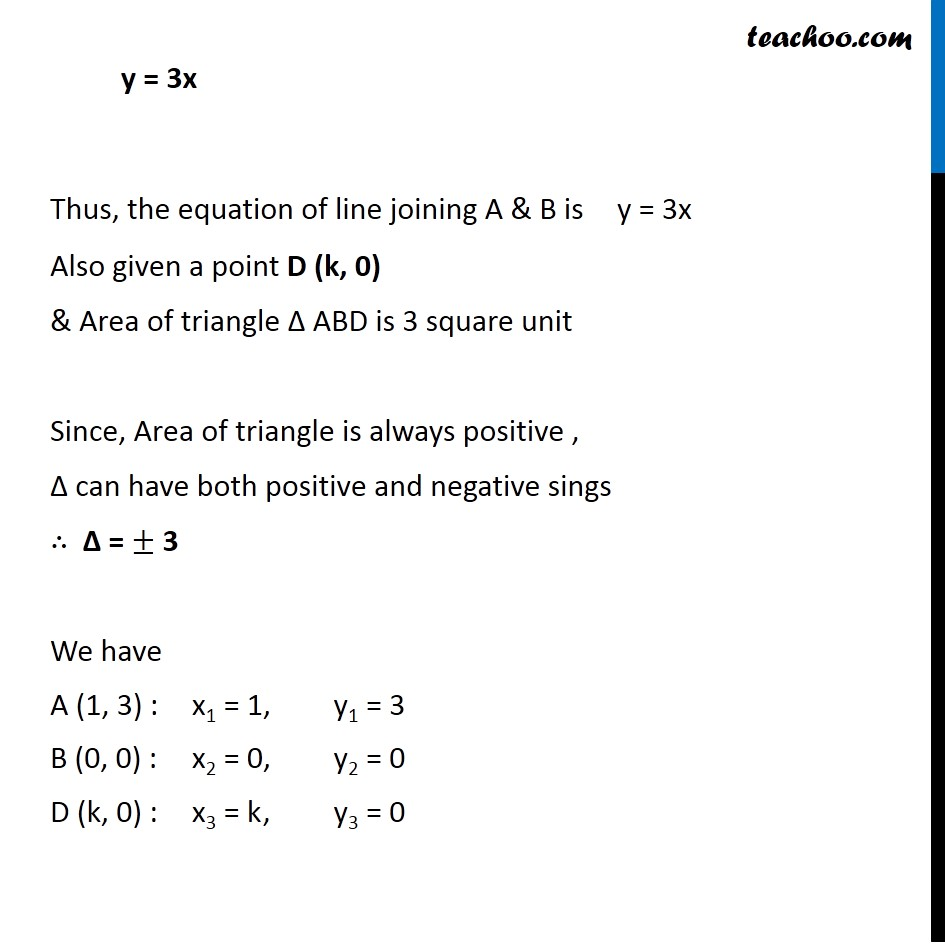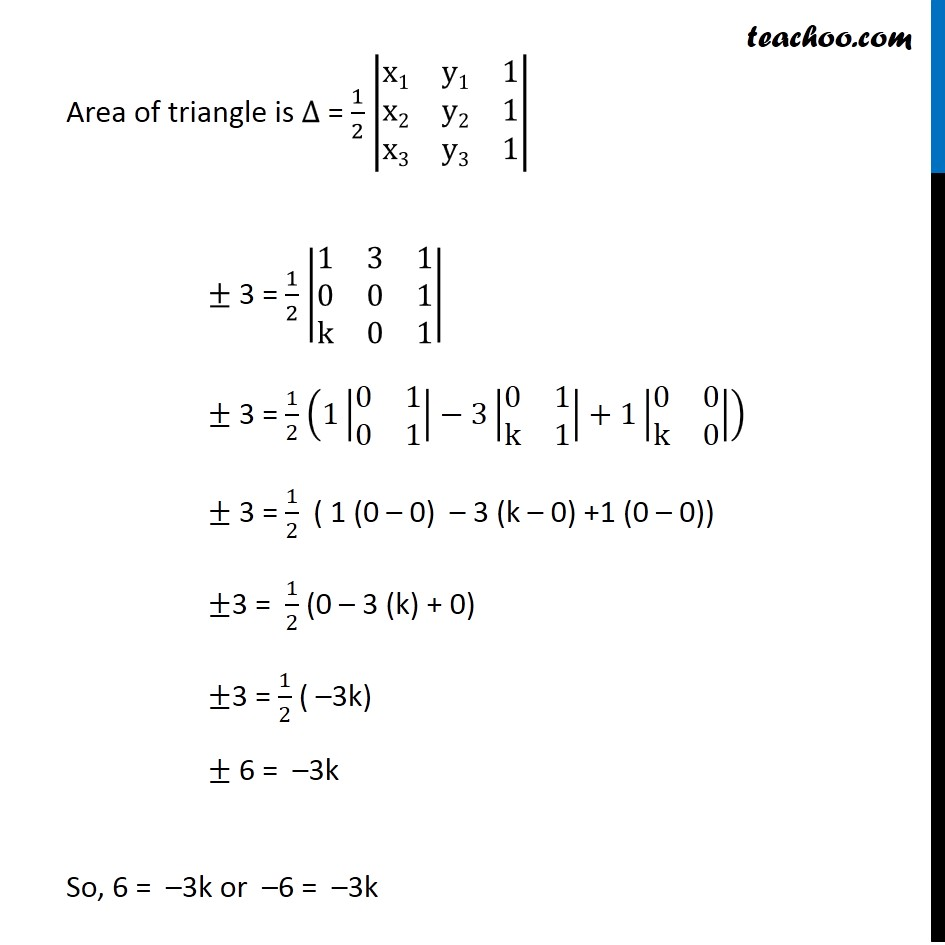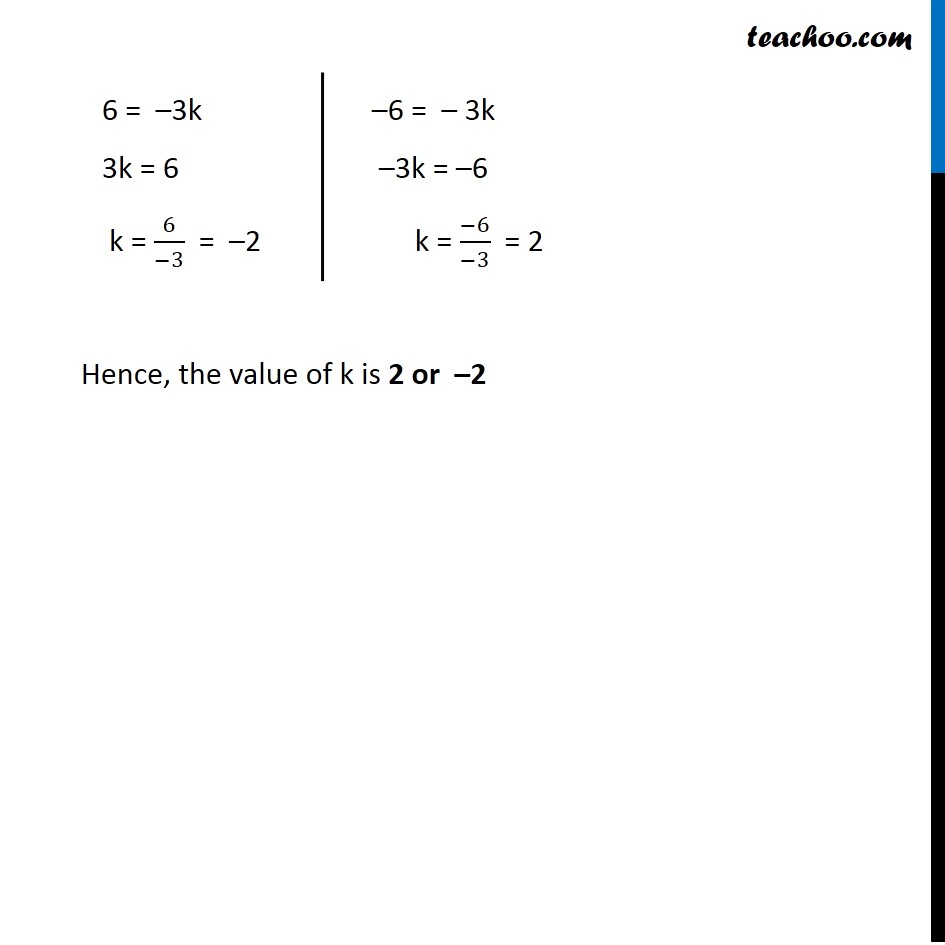1. Chapter 4 Class 12 Determinants (Term 1)
2. Concept wise
3. Equation of line using determinant

Transcript

Example 18 Find the equation of the line joining A(1, 3) and B(0, 0) using determinants and find k if D(k, 0) is a point such that area of triangle ABD is 3 sq units. Equation of line Let L be the line joining the A(1, 3) & B(0, 0) Let (x, y) be the third point on line Since all the three points lie on the same line, they do not from a triangle Hence, Area of triangle = 0 Thus, ∆ = 0 We know that Area of triangle is given by ∆ = 1/2 |■8(x1&y1&1@x2&y2&1@x3&y3&1)| Here, ∆ = 0 x1 = x , y1 = y x2 = 1 , y2 = 3 x3 = 0 , y3 = 0 3 points collinear Area of triangle = 0 Area of triangle ≠ 0 Putting values 0 = 1/2 |■8(𝑥&𝑦&1@1&3&1@0&0&1)| 0 = 1/2 (𝑥|■8(3&1@0&1)|−𝑦|■8(1&1@0&1)|+1|■8(1&3@0&0)|) 0 = 1/2 ( x (3 – 0) – y (1 – 0) +1 (0 – 0)) 0 = 1/2 (x (3) – y (1) + 0) 0 = 1/2 (3x – y) 2 × 0 = 3x – y 0 = 3x – y 3x – y = 0 y = 3x Thus, the equation of line joining A & B is y = 3x Also given a point D (k, 0) & Area of triangle ∆ ABD is 3 square unit Since, Area of triangle is always positive , ∆ can have both positive and negative sings ∴ ∆ = ± 3 We have A (1, 3) : x1 = 1, y1 = 3 B (0, 0) : x2 = 0, y2 = 0 D (k, 0) : x3 = k , y3 = 0 Area of triangle is ∆ = 1/2 |■8(x1&y1&1@x2&y2&1@x3&y3&1)| ± 3 = 1/2 |■8(1&3&1@0&0&1@k&0&1)| ± 3 = 1/2 (1|■8(0&1@0&1)|−3|■8(0&1@k&1)|+1|■8(0&0@k&0)|) ± 3 = 1/2 ( 1 (0 – 0) – 3 (k – 0) +1 (0 – 0)) ±3 = 1/2 (0 – 3 (k) + 0) ±3 = 1/2 ( –3k) ± 6 = –3k So, 6 = –3k or –6 = –3k 6 = –3k 3k = 6 k = 6/(−3) = –2 –6 = – 3k –3k = –6 k = (−6)/(−3) = 2 Hence, the value of k is 2 or –2